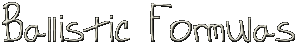# Shotgun Recoil Energy, Impulse & Velocity Calculator

Usage: Select the UOM system, that is English (lbs & fps) or Metric (kgs & meters), by default english is taken.
Type in the appropriate values & click the Calculate button. Change of the UOM system setting causes recalculation of the given data. UOM system change converts the entered data to the corresponding UOM system, so you can use it as a converter too :)

``` ```

```The formulas used for calculations are: RI=(SM+WM+PC*CM)*MV/g*mcoef - for Recoil Impulse; RV=g*RI/GM - for Recoil Velocity; RE=GM*RV2/(2*g) - for Recoil Energy; SM is shot mass in grains or gramms, SM > 0;* WM is wad mass in grains or gramms, WM > 0;* MV is the muzzle velocity in ft/sec or m/sec, MV > 0;* GM is the gun mass in lb or kg, GM > 0;* CM is the charge mass in grains grains or gramms, CM > 0;* g is the gravitaional constant, 32.17 or 9.81; PC is the coefficient that equals 1.5 (I assume the same value for the metric system, might be wrong); mcoef is 7000(number of grains in lb) for english system & 1000(gramms in kg) for Metric system. * - Only first two decimal digits are used. ```